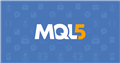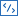# mql5 invalid stops - error

Hi All, as a newbie with mt5, I tried to write an ea an get error saying invalid stops, here is the code of my ea for opening position:

```void TryCreatePosition(ENUM_ORDER_TYPE type)
{
double sl = 0, tp = 0;
CalcSLTP(type, pOpen, sl, tp);
if(sl > 0)
sl = NormalizeDouble(sl, _Digits);
if(tp > 0)
tp = NormalizeDouble(tp, _Digits);
double calc_lot = 0;
if(LM == LM_Fixed)
calc_lot = LOT;
else
{
double pips = MathAbs(pOpen  - sl) / SymbolInfoDouble(_Symbol, SYMBOL_POINT);
if(pips == 0 || tick_value == 0)
{
ea_error="Can't calculate lotsize!(points=0)";
return;
}
double balance = AccountInfoDouble(ACCOUNT_BALANCE);
double riskMoney = balance * (RiskPercentage / 100.0);
calc_lot = riskMoney / pips / tick_value;
double lotStep = SymbolInfoDouble(_Symbol, SYMBOL_VOLUME_STEP);
calc_lot = MathRound(calc_lot / lotStep) * lotStep;
}
if(calc_lot==0)
{
ea_error="Can't calculate lotsize!";
return;
}
string c_msg="Order opened with timeframe ("+ GetTimeframeString() +")";
//bool ret = trade.PositionOpen(_Symbol, type, calc_lot, pOpen, sl, tp, c_msg);
for(int i=0; i<5; i++)
{
bool ret = trade.PositionOpen(_Symbol, type, calc_lot, pOpen, 0, tp, c_msg);
if(ret)
{
last_date= iTime(_Symbol, _Period, 0);
Notify(
+"\nSymbol: " + _Symbol
+"\nLotSize: " + (string)calc_lot
);
}
else
{
Print(ea_error);
}
}
}```Documentation on MQL5: Constants, Enumerations and Structures / Environment State / Symbol Properties
• www.mql5.com
Symbol Properties - Environment State - Constants, Enumerations and Structures - MQL5 Reference - Reference on algorithmic/automated trading language for MetaTrader 5
Files:

Print your SL, PT and prices of the order, bid and ask and check whether they all are placed well and have the rquested distance acc. to the specifications <= this check should be done by your EA before it sends any order!

Ambinintsoa Nathan:

Hi All, as a newbie with mt5, I tried to write an ea an get error saying invalid stops, here is the code of my ea for opening position:

Please insert the code correctly: when editing a message, press the buttonand paste your code into the pop-up window. (The first time I corrected your message)

Carl Schreiber #:
Print your SL, PT and prices of the order, bid and ask and check whether they all are placed well and have the rquested distance acc. to the specifications <= this check should be done by your EA before it sends any order!

Thanks Carl,

The thing is: stop loss = 0, I don't want to set a stop loss.

Ambinintsoa Nathan #:

Thanks Carl,

The thing is: stop loss = 0, I don't want to set a stop loss.

Here is the stoploss parameters
Files:

Ambinintsoa Nathan #:
Here is the stoploss parameters

here it is;

```input   group "Trading Settings"

input   ENUM_LOT_MOD LM = LM_Fixed;             //LotSize Type
input   double          LOT = 0.2;                //LotSize: Fixed
input   double          RiskPercentage = 1;        //LotSize: % of Balance
input   double             StoplossInPips = 0;     //Stoploss(in Points, 0=no stoploss)
input   double             TakeprofitInPips = 2.5;   //Takeprofit(in Points, 0=Take profit)
input    ENUM_TIMEFRAMES TF_W=PERIOD_M1;//Working Timeframe
input   bool TF_H_E=true;//Use Higher Timeframe
input    ENUM_TIMEFRAMES TF_H=PERIOD_M5;//Higher Timeframe
int           Slippage                    = 50;          //Slippage
input   bool        CloseIfOppositeSignal            = true;            //Close trades when opposite signal
input   bool          ClosedCandle                    = true;           //Open trades when candle closed

```
Reason: